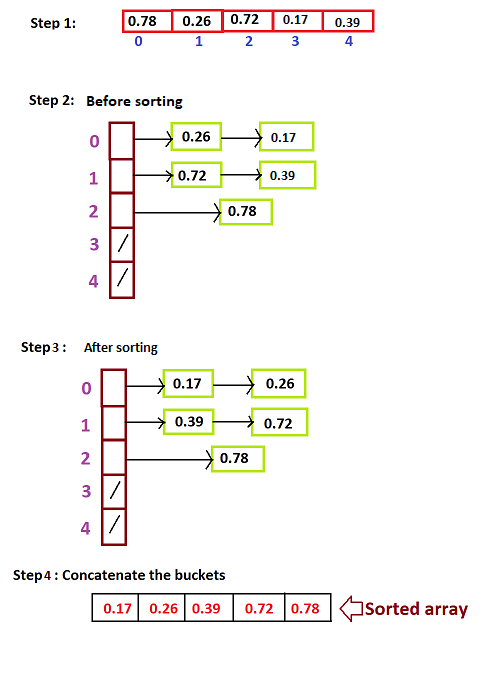×

Search anything:

# Bucket Sort Algorithm

#### Sorting Algorithms Algorithms bucket sortGet this book -> Problems on Array: For Interviews and Competitive Programming

Reading time: 30 minutes | Coding time: 10 minutes

Bucket sort is a comparison sort algorithm that works by distributing the elements of an array into a number of buckets and then each bucket is sorted individually using a separate sorting algorithm or by applying the bucket sort algorithm recursively.This algorithm is mainly useful when the input is uniformly distributed over a range.

### Algorithm

1. Create an empty array of size n(n empty buckets).
2. Loop through the original array and put each array element in a “bucket”.
3. Sort each of the non-empty buckets using insertion sort.
4. Visit the buckets in order and put all elements back into the original array

### Example

Let's take an array A[]={0.78,0.26,0.72,0.17,0.39}. Total number of elements are n=10. So we need to create 5 buckets.
maximum element(max)=0.78
Using the formula: bi = (n)*arr[i]/(max+1), we find out bucket index of each element of the array A[].
For eg: bucket index of 0th element is bi= floor((5)(0.78)/(0.78+1))= 2
Similarly,bucket index of 1st element is bi=0
bucket index of 2nd element is bi=1
bucket index of 3rd element is bi=0
bucket index of 4th element is bi=1
Now, since buckets have been created we need to concatenate all the buckets in the order to get the sorted array.### Pseudocode

Step 1: function bucketSort(array, n) is
Step 1: buckets ← new array of n empty lists
Step 2: M ← the maximum key value in the array
Step 3: for i = 1 to length(array) do
insert array[i] into buckets[floor(array[i] / M * n)]
Step 4: for i = 1 to n do
sort(buckets[i])
Step 5: return the concatenation of buckets, ...., buckets[k]

### Complexity

Worst case time complexity:`Θ(n^2)`
Average case time complexity:`Θ(n+k)`
Best case time complexity:`Θ(n+k)`
Space complexity:`Θ(n+k)`

where n is the number of elements to be sorted and k is the number of buckets

For an upper bound on the worst-case cost, it's sufficient to show that it can't be worse. Assuming that insertion sort takes ≤cn2 steps on n elements, consider the sum

$\phantom{\rule{2em}{0ex}}\sum _{i=1}^{n}c|{B}_{i}{|}^{2}$

it is an upper bound on the cost of sorting all the buckets. For an upper bound on the worst case for bucket sort, maximize this function subject to ∑|Bi|=n (and add the remaining cost, which is O(n) for all inputs).

For a lower bound on the worst-case cost, we have to find an infinite class of actual inputs and show that their cost behaves as claimed. [0,…,0] serves to show an Ω(n2) lower bound.

• C++

### C++

``````
#include <iostream>
#include <algorithm>
#include <vector>
using namespace std;
float findMax(float A[], int n)
{
if (n == 1)
return A;
return max(A[n-1], findMax(A, n-1));
}
void bucketSort(float arr[], int n)
{
float max=findMax(arr,n);
vector<float> b[n];
// 2) Put array elements in different buckets
for (int i=0; i<n; i++)
{
int bi = n*arr[i]/(max+1); // Index in bucket
b[bi].push_back(arr[i]);
}
// 3) Sort individual buckets
for (int i=0; i<n; i++)
sort(b[i].begin(), b[i].end());
// 4) Concatenate all buckets into arr[]
int index = 0;
for (int i = 0; i < n; i++)
for (int j = 0; j < b[i].size(); j++)
arr[index++] = b[i][j];
}
int main()
{
int n;
float arr[n];
for(int i=0;i<n;i++)
cin>>arr[i];
bucketSort(arr, n);
cout << "Sorted array is \n";
for (int i=0; i<n; i++)
cout << arr[i] << " ";
return 0;
}``````

### Applications

Constructing Histograms
One common computation in data visualization and analysis is computing a histogram. For example, n students might be assigned integer scores in some range, such as 0 to 100, and are then placed into ranges or “buckets” based on these scores.

## Question

#### Is Bucket sort an in-place algorithm?

No
Yes
It is definitely not an "in-place" sorting algorithm as it uses the additional space which is as big as the original array.#### Saranya Jena

Student at Kalinga Institute of Industrial Technology, Bhubaneswar

Bucket Sort Algorithm